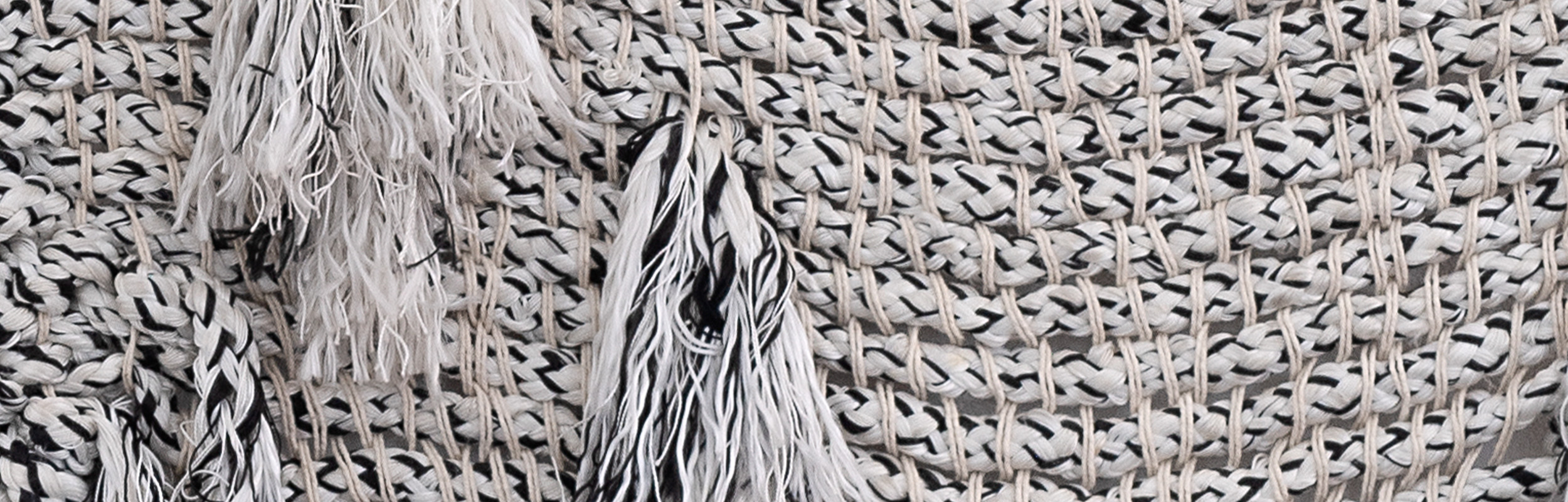## 20th Century & Contemporary Art

Date: 16th Nov, 2023 18:00
Sale number: Jhb Nov 23
Location: Johannesburg

Name: Ms Lucia Boer
Email: lucia@aspireart.net

Name: Sarah Sinisi
Email: sarah@aspireart.net
Telephone: +27 84 568 5639

Estimated at R140,000 - R180,000

Sold for R205,875

Estimated at R180,000 - R240,000

Estimated at R100,000 - R200,000

Sold for R80,062

Estimated at R70,000 - R90,000

Sold for R68,625

Estimated at R60,000 - R80,000

Estimated at R80,000 - R120,000

Estimated at R50,000 - R70,000

Sold for R56,280

Estimated at R50,000 - R70,000

Sold for R137,250

Estimated at R100,000 - R200,000

Sold for R114,375

Estimated at R60,000 - R80,000

Sold for R49,245

Estimated at R40,000 - R60,000

Sold for R35,175

Estimated at R30,000 - R50,000

Sold for R125,812

Estimated at R50,000 - R60,000

Sold for R137,250

Estimated at R50,000 - R60,000

Sold for R80,062

Estimated at R70,000 - R90,000

Sold for R59,475

Estimated at R40,000 - R60,000

Sold for R285,938

Estimated at R250,000 - R350,000

Estimated at R150,000 - R250,000

Estimated at R200,000 - R400,000

Estimated at R100,000 - R150,000

Sold for R183,000

Estimated at R150,000 - R250,000

Estimated at R70,000 - R100,000

Sold for R58,625

Estimated at R50,000 - R70,000

Sold for R251,625

Estimated at R220,000 - R280,000

Estimated at R200,000 - R300,000

Sold for R114,375

Estimated at R100,000 - R150,000

Estimated at R200,000 - R300,000

Sold for R183,000

Estimated at R160,000 - R240,000

Estimated at R40,000 - R60,000

Estimated at R40,000 - R60,000

Sold for R217,312

Estimated at R150,000 - R250,000

Estimated at R40,000 - R60,000

Sold for R58,625

Estimated at R50,000 - R70,000

Sold for R58,625

Estimated at R50,000 - R70,000

Sold for R1,258,125

Estimated at R600,000 - R800,000

Sold for R114,375

Estimated at R100,000 - R200,000

Sold for R514,688

Estimated at R220,000 - R280,000

Estimated at R60,000 - R80,000

Estimated at R300,000 - R500,000

Estimated at R600,000 - R900,000

Sold for R68,625

Estimated at R70,000 - R90,000

Sold for R274,500

Estimated at R250,000 - R350,000

Estimated at R60,000 - R80,000

Estimated at R120,000 - R160,000

Estimated at R200,000 - R300,000

Estimated at R800,000 - R1,200,000

Sold for R960,000

Estimated at R900,000 - R1,200,000

Sold for R320,250

Estimated at R280,000 - R340,000